# Group Statistics in R

A known limitation of R is the substantial overhead to evaluate R expressions. Most workhorse functions hand off quickly to lean pre-compiled routines so this is usually not a problem, but we must be careful with R-level loops such as for, or *pply family1. So long as the number of iterations is small relative to the data size, there is no problem. For example, looping through the columns of a 1,000,000 row by 10 column data frame is fine, but looping through the rows probably won’t be.

A common use case where this limitation is difficult to avoid is the computation of group statistics on data sets with many groups2. This requires a minimum of one R function call for each group, and the additional overhead of splitting the input into groups in R objects.

For example, in:

x   <- c(1, 2, 3, 4, 6, 8, 16)
grp <- c('A', 'B', 'A', 'C', 'A', 'B', 'C')
tapply(x, grp, var)
    A     B     C
6.33 18.00 72.00 

tapply breaks up x according to the groups in grp and applies the var function to each of the resulting pieces of x. Conceptually you can visualize it as follows, with individual colored squares representing vector values and their colors the groups: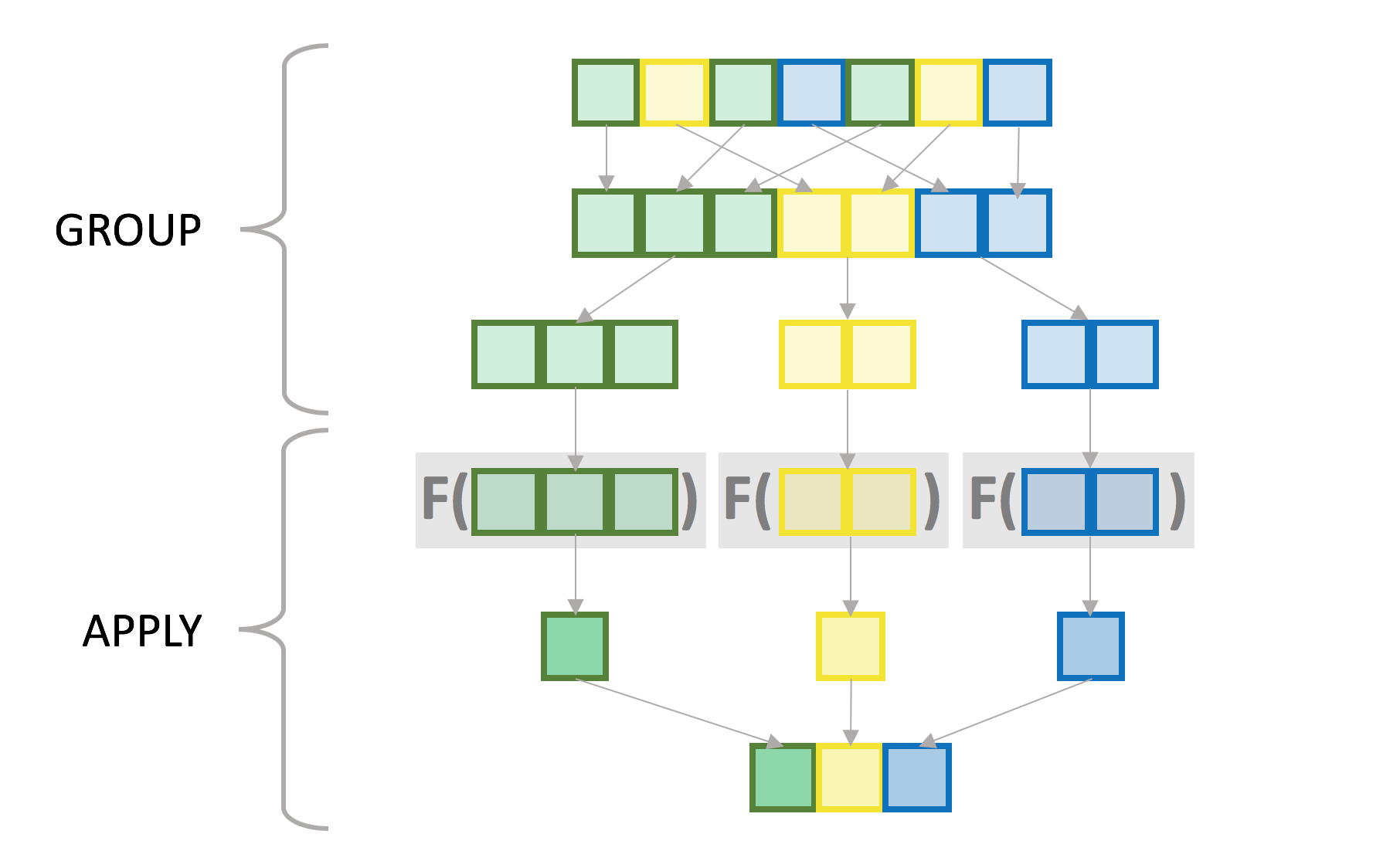While this is not exactly what tapply is doing internally, particularly in the grouping step, the semantics are roughly the same. There is no requirement that the applied function should return a single value per group, although for the purposes of this post we are focusing on that type of function. The result of all this is a vector with as many elements as there are groups and with the groups as the names3.

Let’s try it again with something closer to “real data” size, in this case 10 million values and ~1 million groups4:

RNGversion("3.5.2"); set.seed(42)
n     <- 1e7
n.grp <- 1e6
grp   <- sample(n.grp, n, replace=TRUE)
x     <- runif(n)

system.time(x.grp <- tapply(x, grp, var))
   user  system elapsed
48.933   0.787  50.474

Compare with:

system.time(var(x))
   user  system elapsed
0.061   0.001   0.062

Note: Throughout this post I only show one run from system.time, but I have run the timings several times to confirm they are stable, and I show a representative run5.

Even though both commands are running a similar number of computations in compiled code6, the version that calls the R-level function once is several orders of magnitude faster than the one that calls it for each group.

As alluded to earlier R call overhead is not the only difference between the two examples. tapply must also split the input vector into groups. To evaluate the relative cost of each task, we can separate the tapply call into its grouping (split) and apply components7. split breaks up the input vector into a list with one element for each group:

system.time(x.split <- split(x, grp))
   user  system elapsed
7.124   0.617   7.966
str(x.split[1:3])   # show first 3 groups:
List of 3
$1: num [1:11] 0.216 0.537 0.849 0.847 0.347 ...$ 2: num [1:7] 0.0724 0.1365 0.5364 0.0517 0.1887 ...
$3: num [1:7] 0.842 0.323 0.325 0.49 0.342 ... vapply then collapses each group into one number with the var statistic: system.time(x.grp.2 <- vapply(x.split, var, 0))  user system elapsed 40.001 0.629 41.921 str(x.grp.2)  Named num [1:999953] 0.079 0.0481 0.0715 0.0657 0.0435 ... - attr(*, "names")= chr [1:999953] "1" "2" "3" "4" ... all.equal(c(x.grp), x.grp.2)  TRUE While the var computation accounts for the bulk of the elapsed time, the splitting can become significant with functions that are faster than var8. For example, with sum which is one of the lowest overhead statistics in R, the grouping becomes the limiting element: system.time(vapply(x.split, sum, 0))  user system elapsed 0.605 0.011 0.620 A side-by-side comparison of the two timings makes this obvious. The “group” timing is the same for both functions.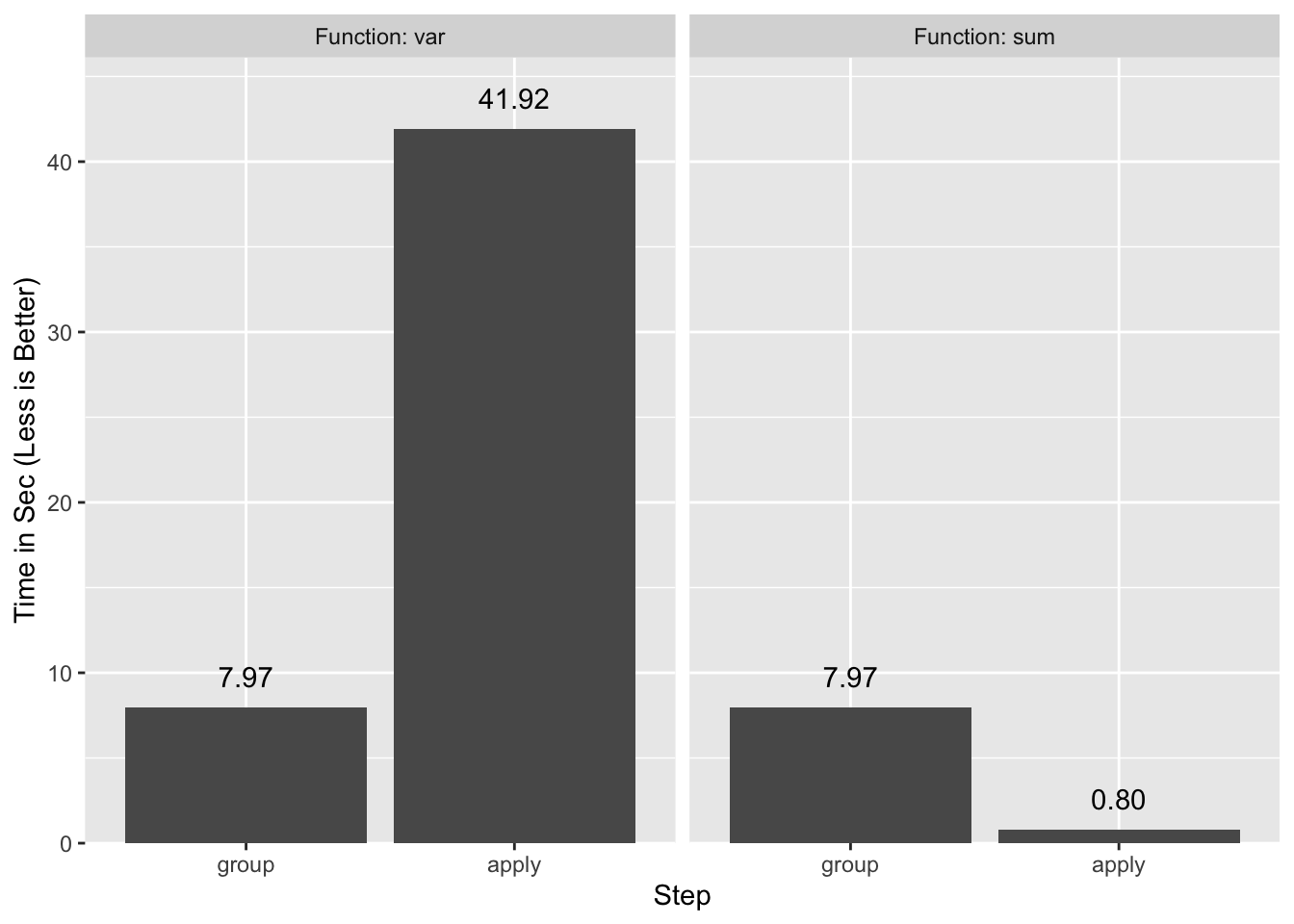tapply is not the only function that computes group statistics in R, but it is one of the simpler and faster ones. # data.table to the Rescue Thankfully the data.table package implements several optimizations to assist with this problem. library(data.table) # 1.12.0 setDTthreads(1) # turn off data.table parallelization Note: I turned off parallelization for data.table as it did not make a significance difference in this particular example, and it muddied the benchmarks9. DT <- data.table(grp, x) DT  grp x 1: 914807 0.116 2: 937076 0.734 --- 9999999: 714619 0.547 10000000: 361639 0.442 In order to compute a group statistic with data.table we use: DT[, var(x), keyby=grp]  grp V1 1: 1 0.0790 2: 2 0.0481 --- 999952: 999999 0.1707 999953: 1000000 0.0667 We use keyby instead of the traditional by because it instructs data.table to group and to order the return table by the groups, which matches what tapply does10. Timings are a different story: system.time(x.grp.dt <- DT[, var(x), keyby=grp][['V1']])  user system elapsed 1.821 0.062 1.909 all.equal(c(x.grp), x.grp.dt, check.attributes=FALSE)  TRUE We’ve reproduced the same results at ~25x the speed. It is not possible to separate out the statistic computation cleanly from the grouping in data.table11, but we can get a rough idea by setting a key on grp. This orders the table by the group, which is most of the overhead of grouping. setkey(DT, grp) # grouping takes advantage of this key DT # notice the table is now ordered by group  grp x 1: 1 0.216 2: 1 0.537 --- 9999999: 1000000 0.180 10000000: 1000000 0.919 What remains is mostly the statistic computation: system.time(DT[, var(x), keyby=grp])  user system elapsed 0.458 0.017 0.477 That step is close to two orders of magnitude faster than with vapply. A summary of the timings: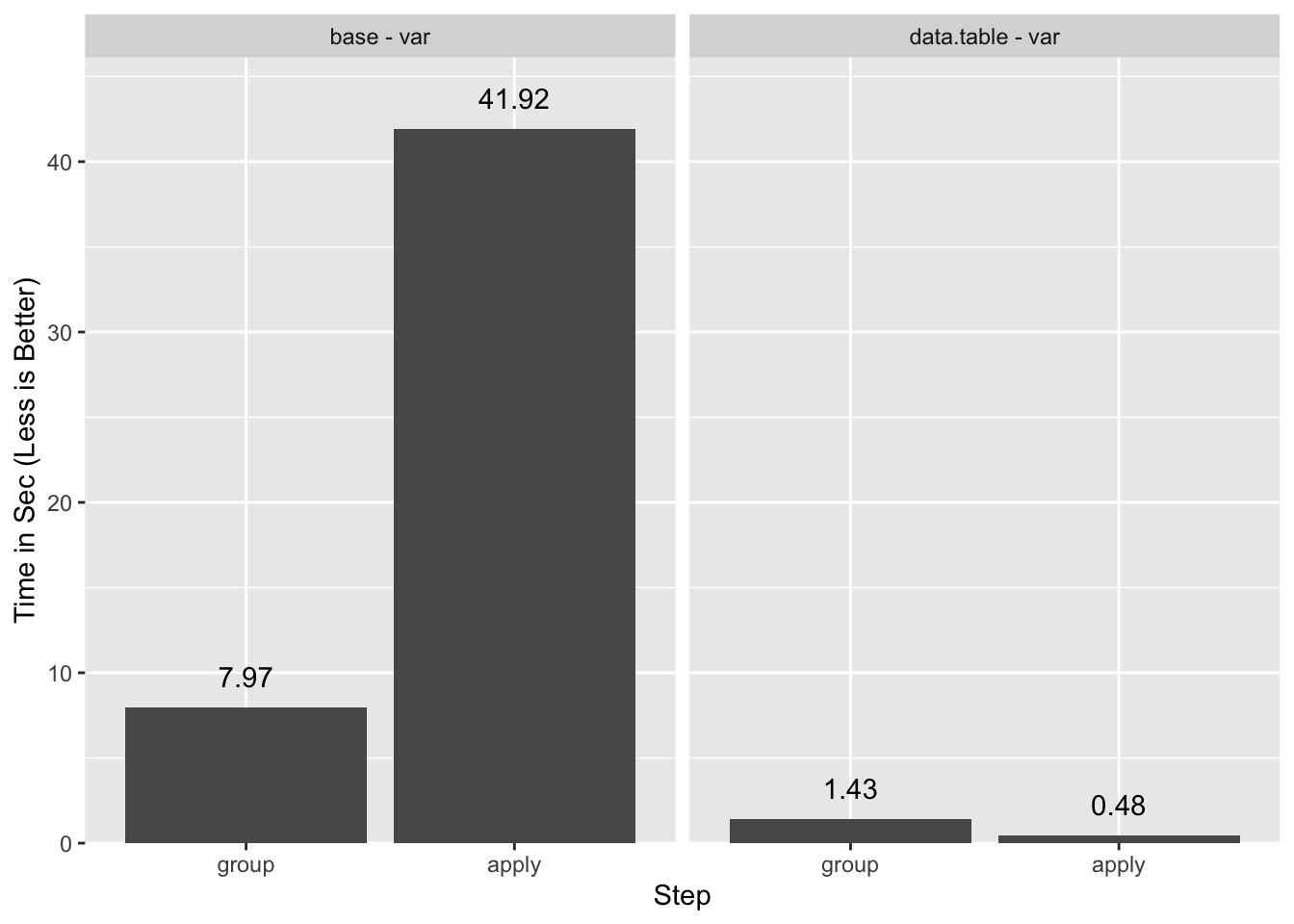# What is this Sorcery? How is it that data.table runs the R var function so much faster than vapply? vapply adds some overhead, but not much as shown when run with the essentially NULL-op unary +12: system.time(vapply(1:1e6, +, 0L))  user system elapsed 0.358 0.006 0.365 It turns out we are being lied to: data.table does not call the R var function ~1 million times. Instead it intercepts the call var(x) and substitutes it with a compiled code routine. This routine computes all the groups without intervening R-level evaluations. The data.table authors call this “GForce” optimization13. The substitution is only possible if there is a pre-existing compiled code equivalent for the R-level function. data.table ships with such equivalents for the “base” R14 functions min, max, mean15, median, prod, sum, sd, var, head, tail16, and [17 as well as for the data.table functions first and last. Additionally, the substitution only happens with the simplest of calls. Adding a mere unary + call causes it to fail: system.time(DT[, +var(x), keyby=grp])  user system elapsed 42.564 0.628 45.394 If you need a different function or a non-trivial expression you’ll have be content with falling back to standard evaluation. You can check whether data.table uses it by setting the verbose option18: options(datatable.verbose=TRUE) DT[, var(x), keyby=grp] Detected that j uses these columns: x Finding groups using uniqlist on key ... 0.113s elapsed (0.045s cpu) Finding group sizes from the positions (can be avoided to save RAM) ... 0.020s elapsed (0.012s cpu) lapply optimization is on, j unchanged as 'var(x)' GForce optimized j to 'gvar(x)' Making each group and running j (GForce TRUE) ... And to confirm that adding the unary + disables “GForce”: DT[, +var(x), keyby=grp] Detected that j uses these columns: x Finding groups using uniqlist on key ... 0.034s elapsed (0.029s cpu) Finding group sizes from the positions (can be avoided to save RAM) ... 0.009s elapsed (0.009s cpu) lapply optimization is on, j unchanged as '+var(x)' GForce is on, left j unchanged Old mean optimization is on, left j unchanged. Making each group and running j (GForce FALSE) # Blood From a Turnip Imagine we wish to compute the slope of a bi-variate least squares regression. The formula is: $\frac{\sum(x_i - \bar{x})(y_i - \bar{y})}{\sum(x_i - \bar{x})^{2}}$ The R equivalent is: slope <- function(x, y) { x_ux <- x - mean(x) uy <- mean(y) sum(x_ux * (y - uy)) / sum(x_ux ^ 2) } In order to use this function with the base split/*pply paradigm we need a little additional manipulation: y <- runif(n) system.time({ id <- seq_along(grp) id.split <- split(id, grp) res.slope.base <- vapply(id.split, function(id) slope(x[id], y[id]), 0) })  user system elapsed 17.745 0.318 18.453 Instead of splitting a single variable, we split an index that we then use to subset each variable. data.table makes this type of computation easy: DT <- data.table(grp, x, y) system.time(res.slope.dt1 <- DT[, slope(x, y), keyby=grp])  user system elapsed 11.501 0.136 11.679 The timings improve over base, but not by the margins we saw previously. This is because data.table cannot use the sum and mean “GForce” counterparts when they are inside another function, or even when they are part of complex expressions. So, what are we to do? Are we stuck writing custom compiled code? Fortunately for us there is one last resort: with a little work we can break up the computation into pieces and take advantage of “GForce”. The idea is to compute intermediate values with mean and sum in simple calls that can use “GForce”, and carry out the rest of the calculations separately. In this case, the rest of the calculations use the operators *, -, /, and ^. These arithmetic operators have the property that their results are the same whether computed with or without groups. It follows that the lack of “GForce” counterparts for the operators is a non-issue. We start by computing $\bar{x}$ and $\bar{y}$: DT <- data.table(grp, x, y) setkey(DT, grp) # more on this in a moment DTsum <- DT[, .(ux=mean(x), uy=mean(y)), keyby=grp] Then we compute the $(x - \bar{x})$ and $(y - \bar{y})$ values by joining (a.k.a merging) our original table DT to the summary table DTsum with the $\bar{x}$ and $\bar{y}$ values. In data.table this can be done by subsetting for rows with another data.table19: DT[DTsum, :=(x_ux=x - ux, y_uy=y - uy)] DT  grp x y x_ux y_uy 1: 1 0.216 0.950 -0.3353 0.5058 2: 1 0.537 0.914 -0.0146 0.4697 --- 9999999: 1000000 0.180 0.589 -0.3681 0.0109 10000000: 1000000 0.919 0.914 0.3711 0.3359 The :=(…) call adds columns to DT with the results of the computations, recycling the $\bar{x}$ and $\bar{y}$ from DTsum. In effect this is equivalent to a join-and-update. We could have achieved the same with the simpler: DT <- data.table(grp, x, y) DT[, :=(ux=mean(x), uy=mean(y)), keyby=grp] While this works, it is much slower because it cannot use “GForce” as data.table does not implement it within :=. So instead we are stuck with the group to DTsum and the join to update DT. If you look back you can see that we used setkey right before computing DTsum20. We did this for two reasons: 1. setkey tells data.table what column to join on when we subset with another data.table as above, and it makes the join fast. 2. Keys can also be used to make grouping faster, so by setting the key early we used it both for the group and the join. The rest is relatively straightforward: DT[, :=(x_ux.y_uy=x_ux * y_uy, x_ux2=x_ux^2)] DTsum <- DT[, .(x_ux.y_uy=sum(x_ux.y_uy), x_ux2=sum(x_ux2)), keyby=grp] res.slope.dt2 <- DTsum[, .(grp, V1=x_ux.y_uy / x_ux2)] And we get the same result as with the base solution: all.equal(res.slope.base, res.slope.dt2[], check.attributes=FALSE)  TRUE Let’s time the whole thing: DT <- data.table(grp, x, y) system.time({ setkey(DT, grp) DTsum <- DT[, .(ux=mean(x), uy=mean(y)), keyby=grp] DT[DTsum, :=(x_ux=x - ux, y_uy=y - uy)] DT[, :=(x_ux.y_uy=x_ux * y_uy, x_ux2=x_ux^2)] DTsum <- DT[, .(x_ux.y_uy=sum(x_ux.y_uy), x_ux2=sum(x_ux2)), keyby=grp] res.slope.dt2 <- DTsum[, .(grp, V1=x_ux.y_uy / x_ux2)] })  user system elapsed 3.278 0.403 3.697 A ~3x improvement over the unoptimized data.table solution, and a ~5x improvement over the base solution. By carefully separating the computations into steps that can use “GForce” and steps that don’t need it to be fast, we are able to get “GForce”-like performance in an application for which that may seem impossible at first blush.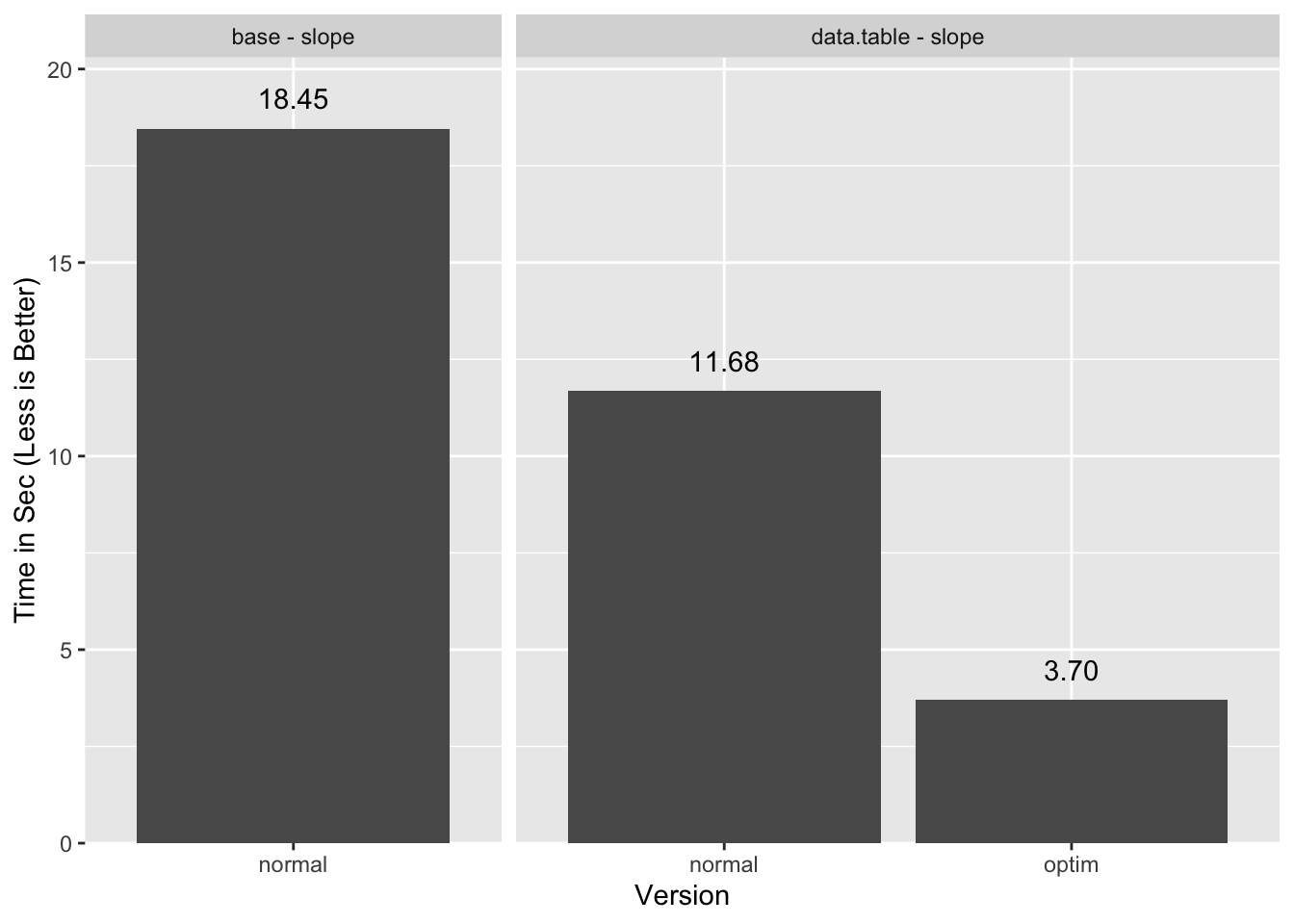Should data.table add “GForce” support for := I estimate the timings could get down to 2.5 seconds or less21 with the cleaner but currently “GForce”-less alternate solution. Hopefully the authors will see it fit to implement this feature. # What About dplyr? Like data.table, dplyr implements a “GForce” style evaluation. dplyr calls this “Hybrid Evaluation”22. For slow functions like var23 this leads to substantial performance improvements over the base solution: library(dplyr) TB <- tibble(grp, x) system.time(TB %>% group_by(grp) %>% summarize(var(x)))  user system elapsed 9.823 0.609 10.639 Performance-wise the “Hybrid Evaluation” component is comparable to data.table’s “Gforce” and is responsible for the improvements over base’s performance: TB.g <- TB %>% group_by(grp) system.time(TB.g %>% summarize(var(x)))  user system elapsed 0.521 0.003 0.527 On the whole dplyr underperforms data.table despite the competitive special evaluation because its grouping step is slower.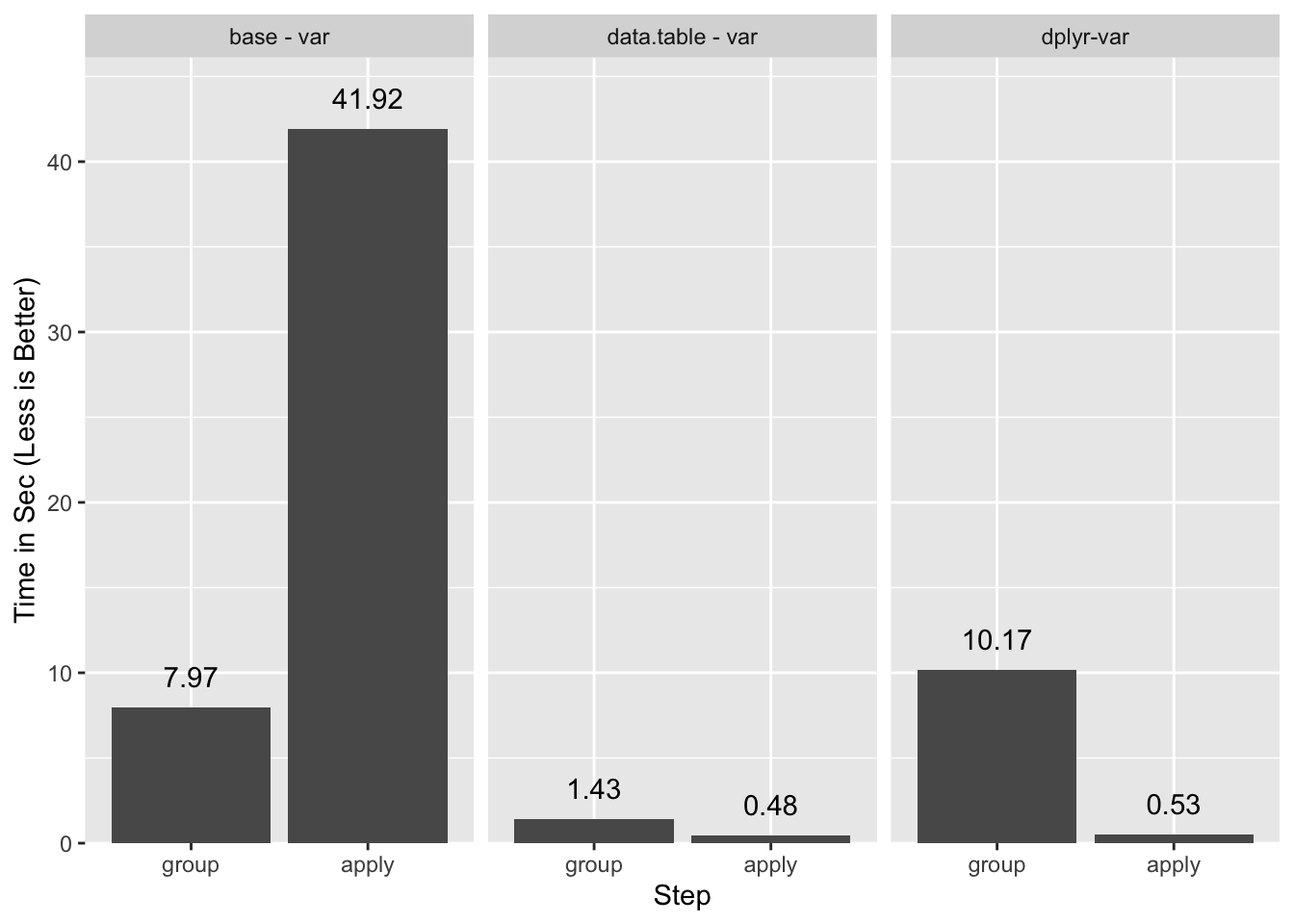Since the dplyr “Hybrid Evaluation” is effective, we should be able to apply the same strategy as with data.table to optimize the computation of slope. Unfortunately it is an uphill battle as shown by the times of the baseline run: TB <- tibble(grp, x, y) system.time(res.dplyr1 <- TB %>% group_by(grp) %>% summarise(slope(x, y)))  user system elapsed 32.65 1.39 34.51 With no attempted optimizations dplyr is ~3x slower than the equivalent data.table call and close to ~2x slower than the base version. Due to the performance impact of group_by the best solution I found is somewhat convoluted. We’ll go through it in steps. First we compute the groups (TB.g) and $\bar{x}$ and $\bar{y}$. We save TB.g as we will re-use it later: TB.g <- TB %>% group_by(grp) TB.u <- TB.g %>% summarize(ux=mean(x), uy=mean(y)) Next is the join and computation of ungrouped stats: TB.s <- TB %>% inner_join(TB.u, by='grp') %>% mutate(x_ux=x-ux, y_uy=y-uy, x_ux.y_uy=x_ux*y_uy, x_ux2=x_ux^2) %>% select(x_ux.y_uy, x_ux2) # A tibble: 10,000,000 x 2 x_ux.y_uy x_ux2 <dbl> <dbl> 1 -0.0488 0.0157 2 0.0401 0.0212 # … with 1e+07 more rows So far this is similar to what we did with data.table except we saved the intermediate statistics into their own table TB.s. We use that in the next step: res.dplyr2 <- bind_cols(TB.g, TB.s) %>% summarise(x_ux.y_uy=sum(x_ux.y_uy), x_ux2=sum(x_ux2)) %>% ungroup %>% mutate(slope=x_ux.y_uy / x_ux2) %>% select(grp, slope) res.dplyr2 # A tibble: 999,953 x 2 grp slope <int> <dbl> 1 1 -0.706 2 2 0.134 # … with 1e+06 more rows all.equal(res.slope.base, res.dplyr2[], check.attributes=FALSE)  TRUE Rather than calling group_by on TB.s at the cost of ~10 seconds, we bind it to TB.g to re-use that tibble’s groups. This only works if the order of rows in TB.g and TB.s is the same. As far as I can tell dplyr does not support a group-ungroup-regroup24 mechanism that would allow us to avoid the explicit re-grouping without having to resort to bind_cols. Let’s time a slightly reworked but equivalent single-pipeline version: system.time({ TB.g <- TB %>% group_by(grp) res.dplyr2a <- TB.g %>% summarise(ux=mean(x), uy=mean(y)) %>% inner_join(TB, ., by='grp') %>% mutate(x_ux=x-ux, y_uy=y-uy, x_ux.y_uy=x_ux*y_uy, x_ux2=x_ux^2) %>% select(x_ux.y_uy, x_ux2) %>% bind_cols(TB.g, .) %>% summarise(x_ux.y_uy=sum(x_ux.y_uy), x_ux2=sum(x_ux2)) %>% ungroup %>% mutate(slope=x_ux.y_uy / x_ux2) %>% select(grp, slope) })  user system elapsed 16.256 0.974 17.591 This is slightly faster than the base solution. I also tried a direct translation of the data.table optimization, but the repeated group_by makes it too slow. I also tried to avoid the join by using mutate instead of summarise for the first grouped calculation but it appears “Hybrid Eval” is not available or is ineffective for mean within mutate, similar to how “GForce” is not available within :=. We can now compare all the timings: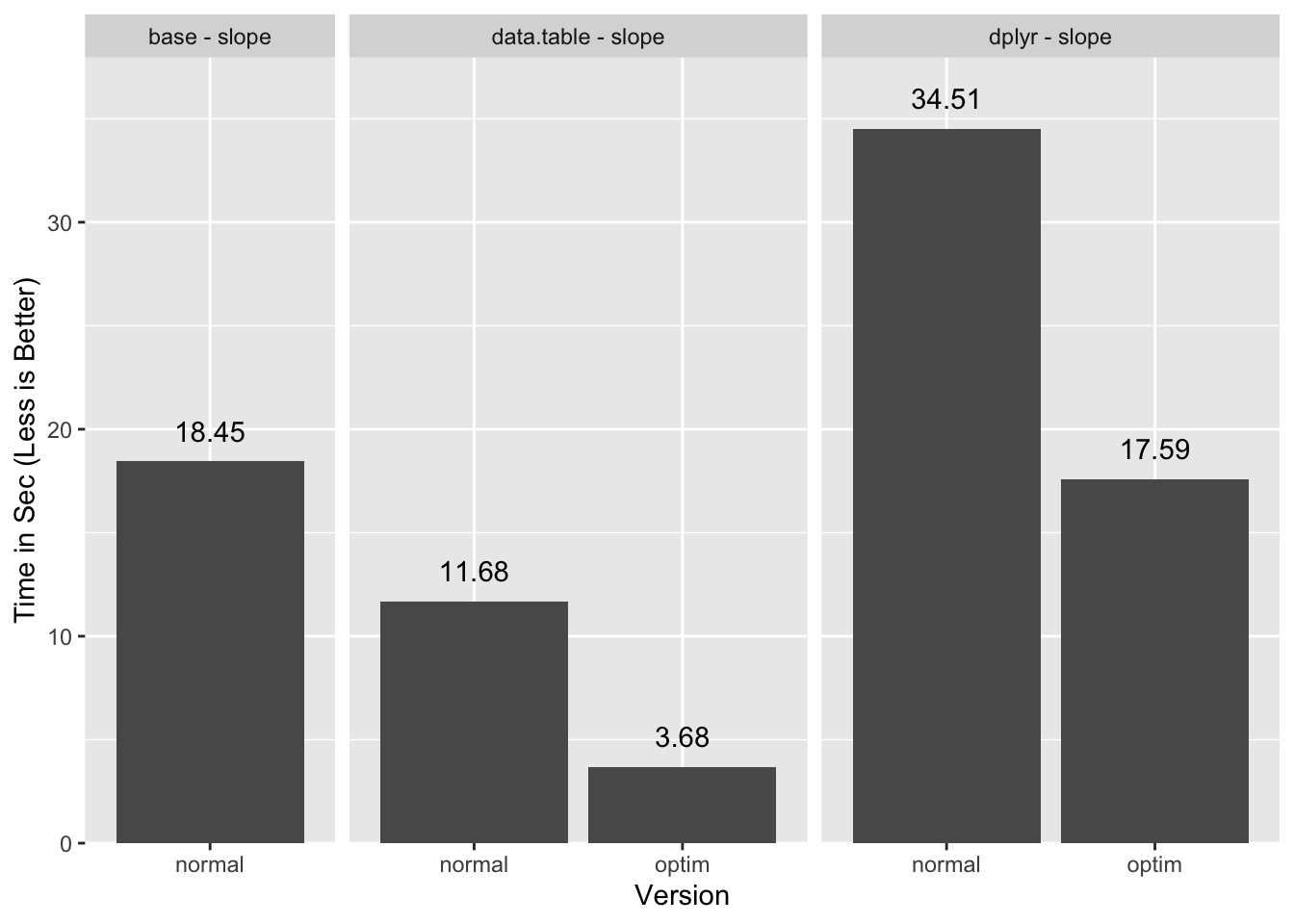data.table benefits a lot more from enabling “GForce” because the group management portion of the execution is small. Since dplyr is less efficient with groups it does not benefit as much, and for this particular task ends up barely faster than base. Certainly this test with many groups and few values per group punishes systems that manage groups and joins with any inefficiency. The data.table team has produced a broader set of benchmarks that you can refer to for some idea of performance for dplyr and data.table under different circumstances. I should point out that “Hybrid Evaluation” is better than “GForce” at respecting the semantics of the calls. For example, dplyr checks that symbols that look like the base summary functions such as mean actually reference those functions. If you do things like mean <- i_am_not_the_mean, dplyr will recognize this and fall back to standard evaluation. Alternatively, if you use mean2 <- base::mean, dplyr will still use “Hybrid Evaluation”. Finally, if a data column actually has a class attribute, dplyr will fall back to standard eval and let dispatch occur. data.table does not handle those cases correctly. This is unlikely to be an issue in typical use cases, but I do like the attention to detail the dplyr team applied here. # Conclusions With a little thought and care we were able to squeeze out some extra performance out of the super optimized data.table package. While a 2-3x performance improvement may not warrant the work required to get it in day-to-day usage, it could come in handy in circumstances where the optimization is written once and re-used over and over. # Appendix ## Acknowledgments ## Updates • 2019-03-11: typos, some blindingly horrible. • 2019-03-12: clarified footnotes, added caveat about “GForce” head/tail. ## Session Info sessionInfo() R version 3.5.2 (2018-12-20) Platform: x86_64-apple-darwin15.6.0 (64-bit) Running under: macOS High Sierra 10.13.6 Matrix products: default BLAS: /Library/Frameworks/R.framework/Versions/3.5/Resources/lib/libRblas.0.dylib LAPACK: /Library/Frameworks/R.framework/Versions/3.5/Resources/lib/libRlapack.dylib locale:  en_US.UTF-8/en_US.UTF-8/en_US.UTF-8/C/en_US.UTF-8/en_US.UTF-8 attached base packages:  stats graphics grDevices utils datasets methods base other attached packages:  dplyr_0.8.0.1 data.table_1.12.0 ggplot2_3.1.0 loaded via a namespace (and not attached):  Rcpp_1.0.0 magrittr_1.5 devtools_1.13.6 tidyselect_0.2.5  munsell_0.5.0 colorspace_1.3-2 R6_2.3.0 rlang_0.3.1  fansi_0.4.0 plyr_1.8.4 tools_3.5.2 grid_3.5.2  gtable_0.2.0 utf8_1.1.4 cli_1.0.1 withr_2.1.2  lazyeval_0.2.1 digest_0.6.18 assertthat_0.2.0 tibble_2.0.1  crayon_1.3.4 purrr_0.2.5 memoise_1.1.0 glue_1.3.0  compiler_3.5.2 pillar_1.3.1 scales_1.0.0 pkgconfig_2.0.2 ## Alternate data.table Solution We can avoid the join by computing the means directly in DT: DT <- data.table(grp, x, y) DT[, :=(ux=mean(x), uy=mean(y)), keyby=grp] DT[, :=(x_ux=x - ux, y_uy=y - uy)] DT[, :=(x_ux.y_uy=x_ux * y_uy, x_ux2=x_ux^2)] DTsum <- DT[, .(x_ux.y_uy=sum(x_ux.y_uy), x_ux2=sum(x_ux2)), keyby=grp] res.slope.dt3 <- DTsum[, .(grp, x_ux.y_uy/x_ux2)] all.equal(res.slope.base, res.slope.dt3[], check.attributes=FALSE)  TRUE This comes at the cost of losing “GForce” for this step as data.table does not currently implement “GForce” within :=: DT[, :=(ux=mean(x), uy=mean(y)), keyby=grp] There happens to be a small performance gain relative to the unoptimized data.table solution, but it is not inherent to this method: DT <- data.table(grp, x, y) system.time({ DT[, :=(ux=mean(x), uy=mean(y)), keyby=grp] DT[, :=(x_ux=x - ux, y_uy=y - uy)] DT[, :=(x_ux.y_uy=x_ux * y_uy, x_ux2=x_ux^2)] DTsum <- DT[, .(x_ux.y_uy=sum(x_ux.y_uy), x_ux2=sum(x_ux2)), keyby=grp] res.slope.dt2 <- DTsum[, .(grp, x_ux.y_uy/x_ux2)] })  user system elapsed 7.749 0.335 8.143 Prior to the implementation of “GForce”, the data.table team introduced an optimization for mean that can still be used in cases where “GForce” is not available. So in this case because data.table can see the mean call, it replaces it with on optimized but still R-level version of mean. There are still ~1 million R-level calls, but because the base mean function is slow due to the R-level S3 dispatch, replacing it with a slimmer R-level equivalent saves about 3 seconds. ## Mutate dplyr Optimization Forsaking “Hybrid Eval” for the first mutate in order to avoid the join, as it is slower than in data.table, makes things a little slower: system.time({ res.dplyr3 <- TB %>% group_by(grp) %>% mutate(ux=mean(x), uy=mean(y)) %>% ungroup %>% mutate(x_ux=x - ux, y_uy=y - uy, x_ux.y_uy = x_ux * y_uy, x_ux2 = x_ux^2) %>% group_by(grp) %>% summarize(sx_ux.y_uy=sum(x_ux.y_uy), sx_ux2=sum(x_ux2)) %>% ungroup %>% mutate(slope= sx_ux.y_uy / sx_ux2) %>% select(grp, slope) })  user system elapsed 30.00 1.03 31.71 ## Join Comparison DT <- data.table(grp, x, y) DT.g <- DT[, .(ux=mean(x), uy=mean(y)), keyby=grp] system.time({ setkey(DT, grp) DT[DT.g] })  user system elapsed 2.305 0.169 2.496 TB <- tibble(grp, x, y) TB.g <- TB %>% group_by(grp) %>% summarise(ux=mean(x), uy=mean(y)) system.time(TB %>% inner_join(TB.g))  user system elapsed 4.888 0.471 5.836 ## Join dplyr Optimization Using the same methodology that we optimized data.table with we get some improvement, but we are still slower than the base method: system.time({ res.dplyr3 <- TB %>% group_by(grp) %>% summarize(ux=mean(x), uy=mean(y)) %>% inner_join(TB, ., by='grp') %>% mutate(x_ux=x-ux, y_uy=y-uy, x_ux.y_uy=x_ux*y_uy, x_ux2=x_ux^2) %>% group_by(grp) %>% summarize(x_ux.y_uy=sum(x_ux.y_uy), x_ux2=sum(x_ux2)) %>% ungroup %>% mutate(slope=x_ux.y_uy / x_ux2) %>% select(grp, slope) })  user system elapsed 26.07 1.45 28.34 all.equal(res.slope.base, res.dplyr3[], check.attributes=FALSE)  TRUE This is because we need to use group_by twice. 1. Even though there is much ado about the differences between explicit loops such as for and while, vs the implicit ones in the *pply family, from a performance perspective they are essentially the same so long as the explicit loops pre-allocate the result vector (e.g. res <- numeric(100); for(i in seq_len(100)) res[i] <- f(i). 2. Anyone who has spent any time answering R tagged questions on Stack Overflow can attest that computing statistics on groups is probably the single most common question people ask. 3. The return value is actually an array, although in this case it only has one dimension. 4. Using a large number of small groups is designed to exaggerate the computational issues in this type of calculation. 5. Normally I would let the knitting process run the benchmarks, but it becomes awkward with relatively slow benchmarks like those in this post. 6. The grouped calculation will require $3(g - 1)$ more divisions than the non-grouped version, where $g$ is the number of groups. However, since there are over 60 arithmetic operations involved in a $n = 10\$ group the overall additional time due to the extra calculations will not dominate even with the relatively expensive division operation.

7. This approximation is semantically “close enough” for the simple example shown here, but will not generalize for more complex inputs.

8. var is particularly slow in R3.5.2 due to the stopifnot calls that it uses. It should become faster in R3.6.0.

9. In particular, we ended up with benchmarks that had user times greater than ellapsed due to the multi-core processing. Anecdotally on my two core system the benchmarks were roughly 20-30% faster with parallelization on. It is worth noting that parallelization is reasonably effective for the gforce step where it close to doubles the processing speed.

10. For more details see the “Aggregation” section of that data.table intro vignette.

11. Setting options(datatable.verbose=TRUE) will actually return this information, but unfortunately in my single threaded testing it seemed to also affect the timings, so I do not rely on it.

12. Even the unary + operator will have some overhead, so not all that time is attributable to vapply.

13. See ?'datatable-optimize' for additional details.

14. By “base” R I mean the packages that are loaded by default in a clean R session, including base, stats, etc.

15. “GForce” mean uses the simpler sum(x) / length(x) calculation, instead of the more precise mean algorithm used by base R. dplyr also uses the more precise algorithm. You can reduce the optimization level on data.table to use a version of mean that aligns with the base version, but that version does not use “GForce” so is substantially slower, although still faster than the base mean function.

16. the “GForce” implementations of head and tail only support head(x, 1) and tail(x, 1) usage, and are effectively equivalent to first and last.

17. For single constant indices e.g. x but not x[1:3] or x[y].

18. Setting options(datatable.verbose=TRUE) will actually return this information, but unfortunately in my single threaded testing it seemed to also affect the timings, so I do not rely on it.

19. Alternatively we can use merge.data.table, although internally that is just a wrapper around [.data.table, though there is the benefit of a familiar interface for those that are used to base::merge.

20. One drawback of using setkey is that it sorts the entire table, which is potentially costly if you have many columns. You should consider subsetting down to the required columns before you use setkey. Another option if you are not looking to join tables is to use setindex.

21. Another benefit of “GForce” in := is that we can then use setindex instead of setkey to accelerate the group computations. The group computation will be a little slower with setindex, but setkey is substantially slower than setindex, so on the whole this should lead to a performance improvement when there is more than one grouping operations.

22. Hybrid eval has evolved a bit between the 0.7.x and 0.8.x releases. The 0.8.0 release notes has good details, as well as the currently unreleased hybrid evaluation vignette. The 0.7.3 vignette has some historical context, and highlights that the original hybrid eval could handle more complex expressions, but that was dropped because the performance improvements did not warrant the additional complexity.

23. var is particularly slow in R3.5.2 due to the stopifnot calls that it uses. It should become faster in R3.6.0.

24. In theory it should be trivial to mark a tibble as ungrouped and have the internal code ignore the groups, and then unmark it later to be treated as grouped again. That’s pure speculation though; I’m not familiar enough with the dplyr internals to be certain.

Brodie Gaslam is a hobbyist programmer based on the US East Coast.